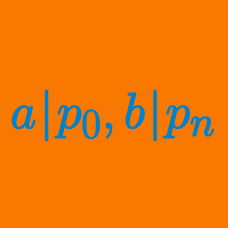Algebra

# Rational Root Theorem: Level 2 Challenges

How many rational roots does $${ x }^{ 1000 }-{ x }^{ 500 }+{ x }^{ 100 }+x+1=0$$ have?

Find the sum of all real roots of

$\ 27x^3+21x+8 = 0$

Given that $$p$$ and $$q$$ are both prime, which of the following answer choices is true about the equation $$px^{ 2 }-qx+q=0?$$

The polynomial $x^3 - 3x^2 + 12x + 16$ has one real root $$r$$ and two complex roots $$w$$ and $$z.$$ What is the value of $$r+ \log_2 \left(w^6 + z^6 \right)$$?

Two of the roots of the equation $ax^3 + bx^2 + cx + d = 0$ are $$2 - \sqrt{3}$$ and $$5$$, where $$a,b,c,d$$ are rational numbers. If the third one is $$m + \sqrt{n}$$ for some integers $$m$$ and $$n$$, find $$m + n$$

×